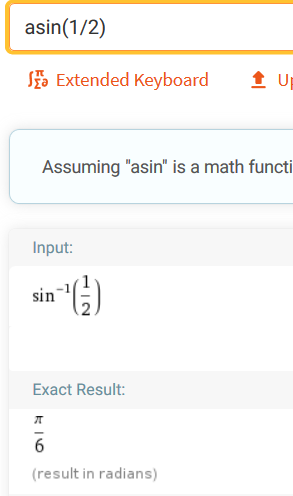# arcsin and other functions

Enrico Gregorio shared this question 5 months ago

There is a discrepancy in how functions are input and output, with Geogebra Graphing Calculator.

If I ask for “arcsin” I get something like “arcsin(<x>)”, which is then printed as sin^-1 in the box.

Now, I object to sin^-1 on mathematical grounds: it is abusive because the sine function has no inverse.

However, I acknowledge that the notation is used.

It would be good if the output could be changed according to the user's preference. But maybe it's already possible: is it?

Particularly strange is the logarithm: if I want the natural logarithm, I need to type in “log”, which prompts for the argument and then it's abruptly transformed into “ln” (another notation I dislike).1

Now it's even worse, because there's no choice of using “log” for the natural logarithm. This should really be left to user preferences.1

Now log is log in base 10, as in pocket calculators. For natural logarithm you must use ln.

About asin that is automatically transformed into sin^-1, this is usual in computational math environment and pocket calculators (see e.g. Wolfram Alpha)1

The fact that calculators or Wolfram use sin^-1 doesn't make the notation correct. It is wrong under mathematical grounds and I wish to see math according to my preferences, not someone else's.